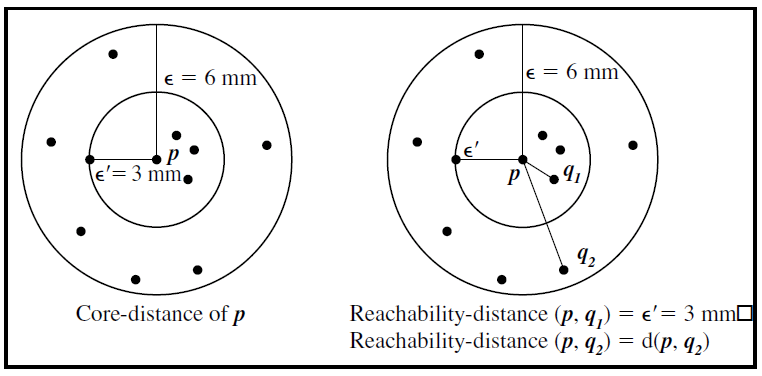2 views

I’m looking for a decent implementation of the OPTICS algorithm in Python. I will use it to form density-based clusters of points ((x,y) pairs).

I'm looking for something that takes in (x,y) pairs and outputs a list of clusters, where each cluster in the list contains a list of (x, y) pairs belonging to that cluster.

by (33.1k points)

Ordering points to identify the clustering structure (OPTICS) is an algorithm for finding density-based clusters in spatial data.For example:

def cluster(order, distance, points, threshold):

clusters = [[]]

points   = sorted(zip(order, distance, points))

splits   = ((v > threshold, p) for i,v,p in points)

for iscluster, point in splits:

if iscluster: clusters[-1].append(point)

elif len(clusters[-1]) > 0: clusters.append([])

return clusters

rd, cd, order = optics(points, 4)

print cluster(order, rd, points, 38.0)